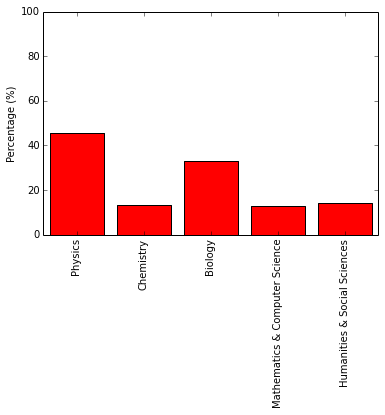In :
import re
import matplotlib.pyplot as plt
import numpy as np
import prettytable
splitter = re.compile(r'(?:[^,(]|$[^)]*$)+')

In :
with open('disciplines.txt') as f:
students = [set(d.strip() for d in splitter.findall(student)) for student in students]

In :
domains = set()
_ = map(domains.update, students)
physics = {'Aerospace Engineering', 'Astrophysics', 'Atmospheric Science',
'Earth sciences (geology, oceanography, meteorology)', 'Engineering (civil, mechanical, chemical)',
'Geography', 'Geological Engineering : Environmental', 'Geological Engineering : Geotechnical',
'Geophysics', 'Hydrogeology', 'Hydrology', 'Physical Oceanography', 'Physics', 'Space sciences',
'astronomy', 'biomedical engineering',}
chemistry = {'Chemical/Geochemical Oceanography', 'Chemistry', 'Materials Science',
'Materials Science and Engineering', 'materials science',}
biology = {'Biological Oceanography', 'Biomechanics', 'Brain and neurosciences', 'Environmental Science',
'Life science (biology, genetics)', 'Life science (ecology, zoology, botany)', 'Medicine',
'Nursing/research',}
mathematics_cs = {'Applied Mathematics', 'Applied math', 'Computer science and electrical engineering',
'Data analysis', 'Mathematics', 'Statistics', 'Tech support', 'data analysis', 'lab tech',
'or support programmer', 'statistics'}
'Humanities and social sciences', 'Law', 'Legal', 'Librarianship', 'Library Science',
'Library Systems', 'Library science', 'Non-profit', 'editing/publishing', 'finance',}
domains = [physics, chemistry, biology, mathematics_cs, human_social]
domain_labels = ["Physics", "Chemistry", "Biology", "Mathematics & Computer Science", "Humanities & Social Sciences"]


Note that the counts calculation may have a sum greater than the total number of students since students may select more than one domain.

In :
counts = np.zeros(5, int)
for i, domain in enumerate(domains):
for student in students:
counts[i] += bool(student & domain)

In :
ndat = len(domains)
dat = 100 * np.asarray(counts, float) / len(students)
fig = plt.figure(1)
p = plt.subplot(1, 1, 1)
plt.bar(range(ndat), dat, align='center', fc='r')
plt.axis([-0.5, ndat - 0.5, 0, 100])
plt.xticks(range(ndat), domain_labels, rotation=90)
plt.ylabel("Percentage (%)")
plt.savefig("students_by_domain.svg", bbox_inches="tight")
plt.show()
plt.close()In :
tab = prettytable.PrettyTable(["Domain", "Percentage (%)"])
for x, y in zip(domain_labels, dat):

In :
print tab.get_string()

+--------------------------------+----------------+
|             Domain             | Percentage (%) |
+--------------------------------+----------------+
|            Physics             |     45.75      |
|           Chemistry            |     13.40      |
|            Biology             |     33.01      |
| Mathematics & Computer Science |     13.07      |
|  Humanities & Social Sciences  |     14.38      |
+--------------------------------+----------------+

In :
print tab.get_html_string()

<table border="1">
<tr>
<th>Domain</th>
<th>Percentage (%)</th>
</tr>
<tr>
<td>Physics</td>
<td>45.75</td>
</tr>
<tr>
<td>Chemistry</td>
<td>13.40</td>
</tr>
<tr>
<td>Biology</td>
<td>33.01</td>
</tr>
<tr>
<td>Mathematics &amp; Computer Science</td>
<td>13.07</td>
</tr>
<tr>
<td>Humanities &amp; Social Sciences</td>
<td>14.38</td>
</tr>
</table>

In :